Search

Graphing Logarithmic Functions: Intro (page 1 of 3)

By nature of the logarithm, most log graphs tend to have the same shape, looking similar to a square-root graph:

 y = sqrt(x) y = log2(x)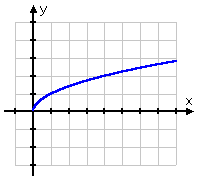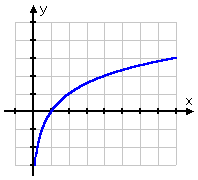The graph of the square root starts at the point (0, 0) and then goes off to the right. On the other hand, the graph of the log passes through (1, 0), going off to the right but also sliding down the positive side of the y-axis. Remembering that logs are the inverses of exponentials, this shape for the log graph makes perfect sense: the graph of the log, being the inverse of the exponential, would just be the "flip" of the graph of the exponential:

 y = 2x y = log2(x)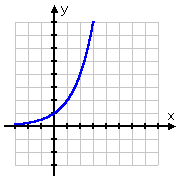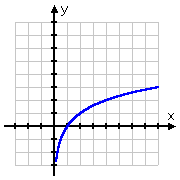comparison of the two graphs, showing the inversion line in red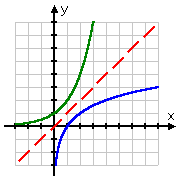It is fairly simple to graph exponentials. For instance, to graph y = 2x, you would just plug in some values for x, compute the corresponding y-values, and plot the points. But how do you graph logs? There are two options. Here is the first:   Copyright © Elizabeth Stapel 2002-2011 All Rights Reserved

• Graph y = log2(x).

In order to graph this "by hand", I need first to remember that logs are not defined for negative x or for x = 0. Because of this restriction on the domain (the input values) of the log, I won't even bother trying to find y-values for, say, x = 3 or x = 0. Instead, I'll start with x = 1, and work from there, using the definition of the log.

• Since 20 = 1, then log2(1) = 0, and (1, 0) is on the graph.
• Since 21 = 2, then log2(2) = 1, and (2, 1) is on the graph.
• Since 3 is not a power of 2, then log2(3) will be some messy value. So I won't bother with graphing x = 3.
• Since 22 = 4, then log2(4) = 2, and (4, 2) is on the graph.
• Since 5, 6, and 7 aren't powers of 2 either, I'll skip them and move up to x = 8.
• Since 23 = 8, then log2(8) = 3, so (8, 3) is on the graph.
• The next power of 2 is 16: since 24 = 16, then log2(16) = 4, and (16, 4) is on the graph.
• The next power of 2, x = 32, is too big for my taste; I don't feel like drawing my graph that wide, so I'll quit at x = 16.
• The above gives me the point (1, 0) and some points to the right, but what do I do for x-values between 0 and 1? For this interval, I need to think in terms of negative powers and reciprocals. Just as the left-hand "half" of the exponential function had few graphable points (the rest of them being too close to the x-axis), so also the bottom "half" of the log function has few graphable points, the rest of them being too close to the y-axis. But I can find a few:

Since 21 = 1/2 = 0.5, then log2(0.5) = 1, and (0.5, 1) is on the graph.

Since 22 = 1/4 = 0.25, then log2(0.25) = 2, and (0.25, 2) is on the graph.

Since 23 = 1/8 = 0.125, then log2(0.125) = 3, and (0.125, 3) is on the graph.

The next power of 2 (as x moves in this direction) is 1/16 = 24, but the x-value for the point (0.0625, 4) seems too small to bother with, so I'll quit with the points I've already found.

 Listing these points gives me my T-chart: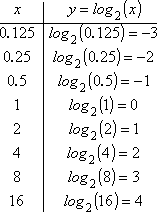Drawing my dots and then sketching in the line (remembering not to go to the left of the y-axis!), I get this graph: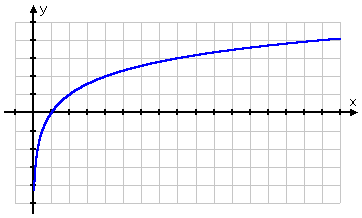Top  |  1 | 2 | 3  |  Return to Index  Next >>

 Cite this article as: Stapel, Elizabeth. "Graphing Logarithmic Functions: Intro." Purplemath. Available from     https://www.purplemath.com/modules/graphlog.htm. Accessed [Date] [Month] 2016

Study Skills Survey

Tutoring from Purplemath
Find a local math tutor